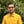Trusted answers to developer questions
Trusted Answers to Developer Questions

Related Tags

ruby
math
communitycreator

# What is Math.sinh() in Ruby?Harris Amjad

Grokking Modern System Design Interview for Engineers & Managers

Ace your System Design Interview and take your career to the next level. Learn to handle the design of applications like Netflix, Quora, Facebook, Uber, and many more in a 45-min interview. Learn the RESHADED framework for architecting web-scale applications by determining requirements, constraints, and assumptions before diving into a step-by-step design process.

The sinh() function in Ruby calculates and returns the hyperbolic sine of a number. To be more specific, it returns the hyperbolic sine of a number in radians.

The figure below shows the mathematical representation of the sinh() function.

Figure 1: Mathematical representation of the hyperbolic sine function

Note: This function is present in the Math module.

## Syntax

sinh(num)


## Parameter

This function requires a number that represents an angle in radians as a parameter.

The following formula converts degrees to radians.

radians = degrees * ( pi / 180.0 )


## Return value

sinh() returns a number’s hyperbolic sine (radians), which is sent as a parameter.

## Example

#positive number in radiansputs "The value of sinh(2.3) : ",  Math.sinh(2.3)#negative number in radiansputs "The value of sinh(-2.3) : ",  Math.sinh(-2.3)#converting the degrees angle into radians and then applying sinh()#degrees = 90#PI = 3.14159265result = 90.0 * (Math::PI / 180.0)puts "The value of sinh(#{result}) : ",  Math.sinh(result)

RELATED TAGS

ruby
math
communitycreator

CONTRIBUTORHarris Amjad

Grokking Modern System Design Interview for Engineers & Managers

Ace your System Design Interview and take your career to the next level. Learn to handle the design of applications like Netflix, Quora, Facebook, Uber, and many more in a 45-min interview. Learn the RESHADED framework for architecting web-scale applications by determining requirements, constraints, and assumptions before diving into a step-by-step design process.

Keep Exploring

Learn in-demand tech skills in half the time